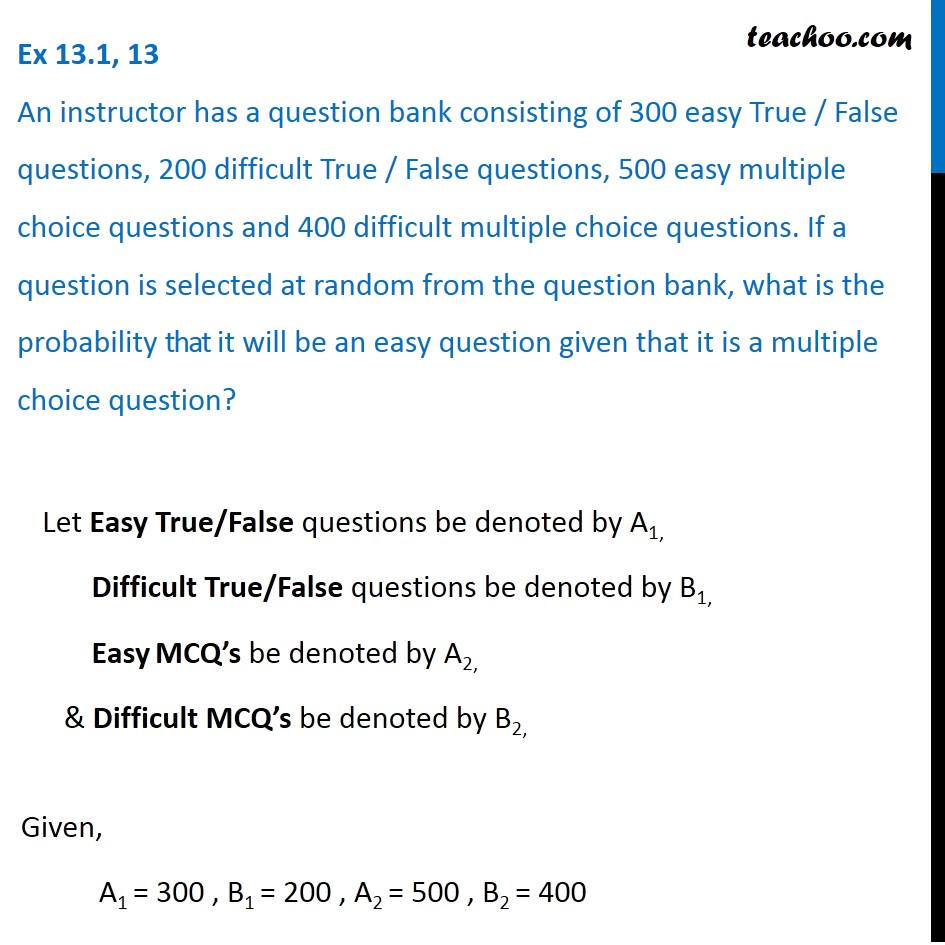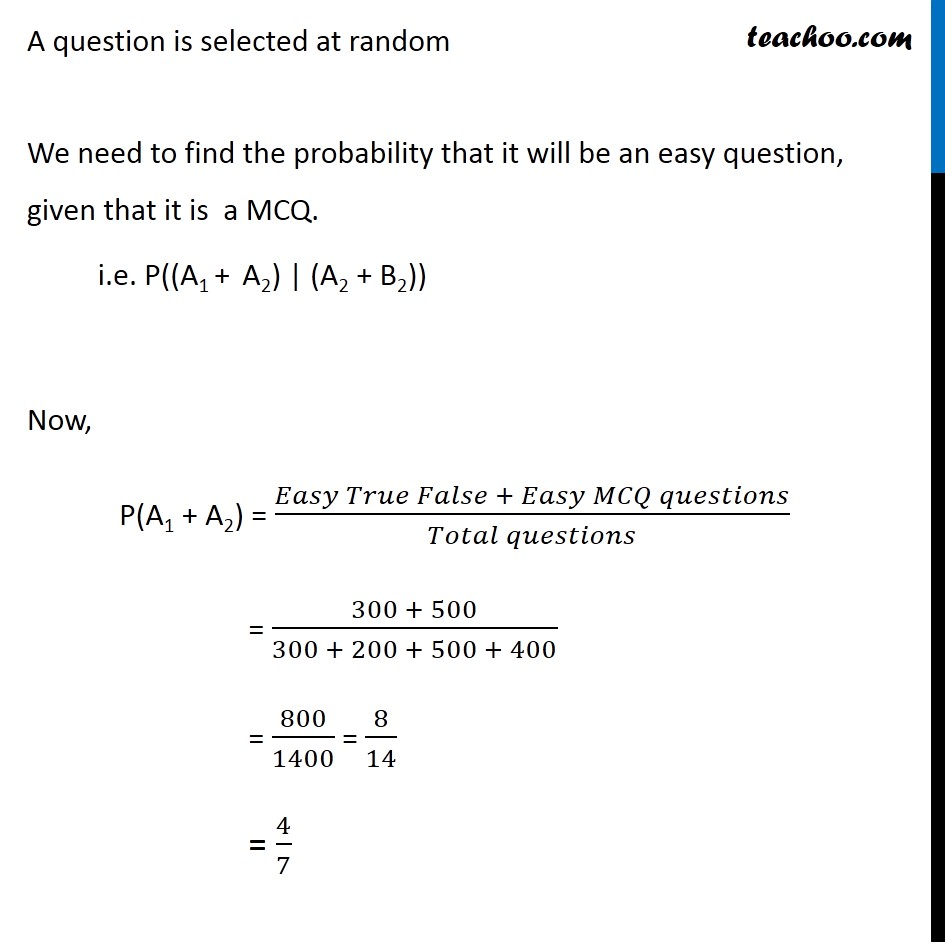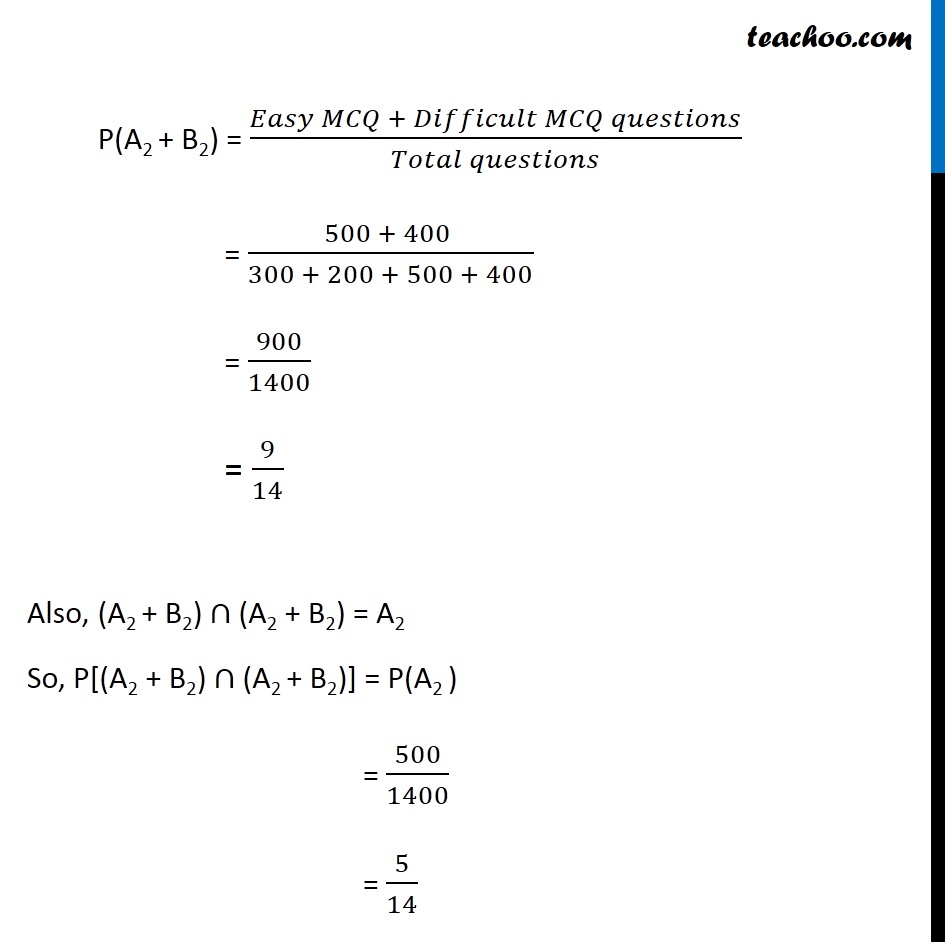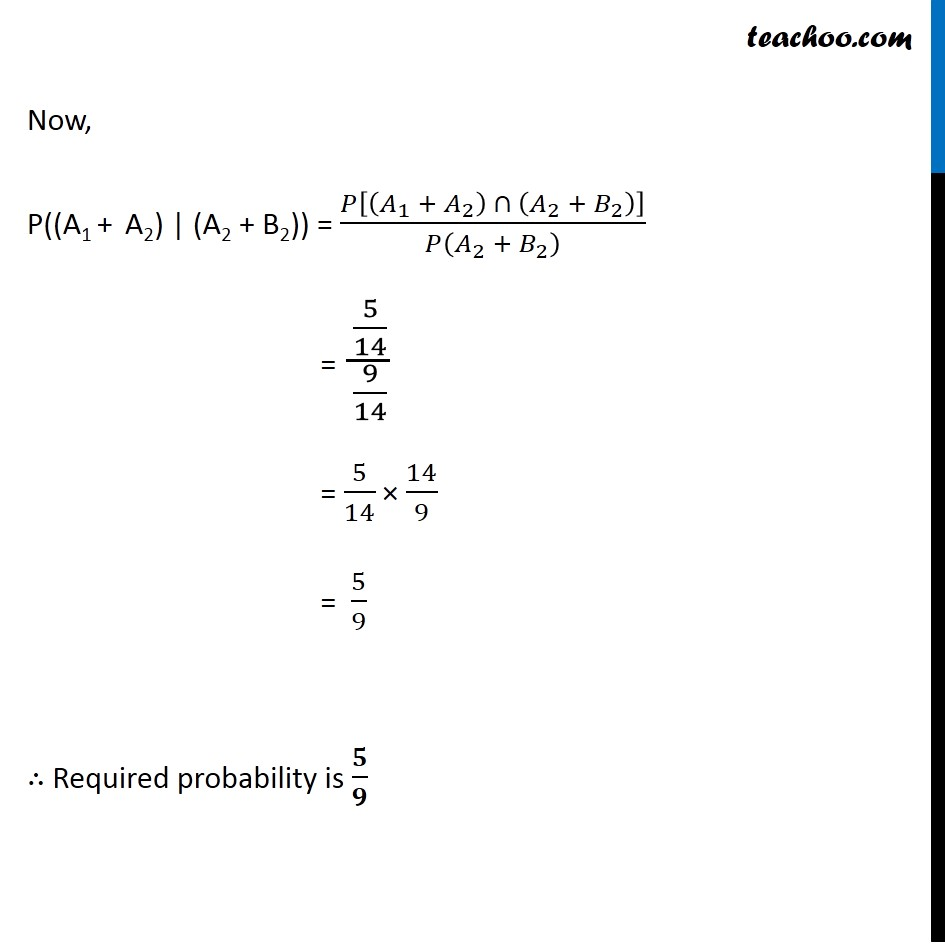Ex 13.1

Chapter 13 Class 12 Probability
Serial order wiseLearn in your speed, with individual attention - Teachoo Maths 1-on-1 Class

### Transcript

Ex 13.1, 13 An instructor has a question bank consisting of 300 easy True / False questions, 200 difficult True / False questions, 500 easy multiple choice questions and 400 difficult multiple choice questions. If a question is selected at random from the question bank, what is the probability that it will be an easy question given that it is a multiple choice question? Let Easy questions be denoted by E, Difficult questions be denoted by D, MCQ’s be denoted by M & True/False questions be denoted by T Given, E ∩ T = 300 , D ∩ T = 200 , E ∩ M = 500 , D ∩ M = 400 We need to find the probability that it will be an easy question, given that it is a MCQ. i.e. P(E | M) Now, P(E ∩ M) = Probability that question is Easy MCQ = (𝑇𝑜𝑡𝑎𝑙 𝐸𝑎𝑠𝑦 𝑀𝐶𝑄 𝑄𝑢𝑒𝑠𝑡𝑖𝑜𝑛𝑠)/(𝑇𝑜𝑡𝑎𝑙 𝑞𝑢𝑒𝑠𝑡𝑖𝑜𝑛𝑠) = 500/1400 = 𝟓/𝟏𝟒 And, P(M) = (𝑇𝑜𝑡𝑎𝑙 𝑀𝐶𝑄 𝑞𝑢𝑒𝑠𝑡𝑖𝑜𝑛𝑠)/(𝑇𝑜𝑡𝑎𝑙 𝑞𝑢𝑒𝑠𝑡𝑖𝑜𝑛𝑠) = (500 + 400 )/1400 = 900/1400 = 𝟗/𝟏𝟒 Thus, P(E | M) = (𝑃(𝐸 ∩ 𝑀))/(𝑃(𝑀)) = (𝟓/𝟏𝟒)/(𝟗/𝟏𝟒) = 5/14 × 14/9 = 𝟓/𝟗 ∴ Required probability is 𝟓/𝟗